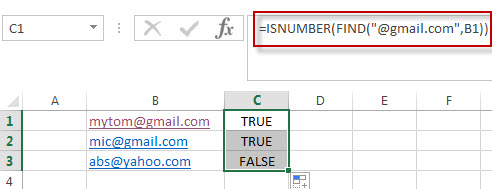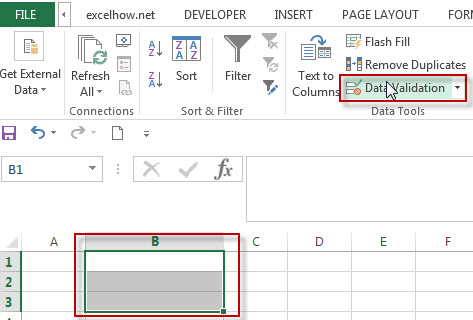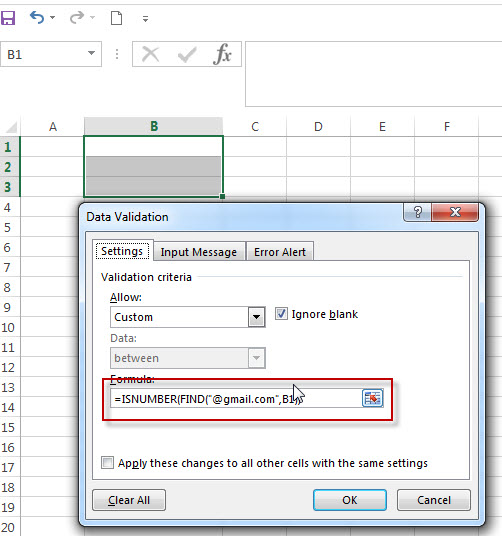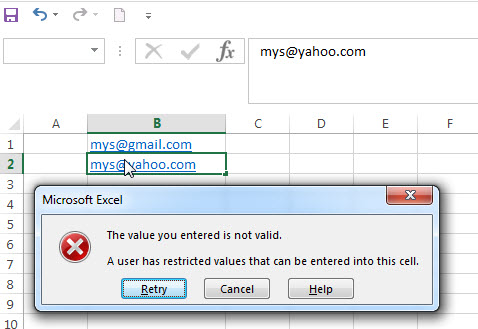# Data Validation for Specified Text only

If you want to check if the values that contain a specified text string in one cell, you can use a combination of the FIND function and ISNUMBER function as a formula in the Data Validation.

## Data Validation for Specified Text only

​Suppose that you want to check that if the values in column B contain the specified text string “@gmail.com”, IF True, then return TRUE, otherwise, it returns FALSE.

You can use the following formula:

`=ISNUMBER(FIND("@gmail.com",B1))`In the above formula, the FIND function will locate the position of the searched text string “@gmail.com” in Cell B1. If the text string is searched, then return the starting position of the searched text string in cell B1. If not, it will return the Excel #VALUE! Error.

The returned results of the FIND function will be as the first argument of the ISNUMBER function. So if the FIND function returns the correct numeric position, then the ISNUMBER function returns TRUE. Otherwise, returns FALSE.

Last, you can add the above formula into Data Validation as rules to B1:B3.When you adds or change the values in range B1:B3, the DATA validation will be triggered.### Related Functions

• Excel Find function
The Excel FIND function returns the position of the first text string (substring) from the first character of the second text string.The FIND function is a build-in function in Microsoft Excel and it is categorized as a Text Function.The syntax of the FIND function is as below:= FIND  (find_text, within_text,[start_num])…
• Excel ISNUMBER function
The Excel ISNUMBER function returns TRUE if the value in a cell is a numeric value, otherwise it will return FALSE.The syntax of the ISNUMBER function is as below:= ISNUMBER (value)…